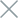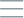• Home
• Tags
• Series
•# Linear Search Algorithm in Java: Exploring and Finding Elements

The Linear Search Algorithm is a simple and fundamental method in Java programming, used to find a specific element within a list or an array. This approach works by traversing each element and comparing it with the search value.

## How the Linear Search Algorithm Works

The Linear Search Algorithm starts from the first element of the list or array. It compares the search value with the value of the current element. If a corresponding value is found, the algorithm returns the position of the element in the list or array. If not found, the algorithm continues moving to the next element and continues the comparison process until the value is found or all elements are traversed.

• Simple and Understandable: This algorithm is easy to implement and understand.
• Works with Any Data Type: Linear search can be applied to any type of list or array data.

• Low Performance: This algorithm requires traversing through all elements in the list or array, which can lead to low performance for large datasets.

## Example and Explanation

Consider an example of using the Linear Search Algorithm to find a specific integer in an integer array in Java.

``````public class LinearSearchExample {
public static int linearSearch(int[] array, int target) {
for (int i = 0; i < array.length; i++) {
if (array[i] == target) {
return i; // Return position if found
}
}
}

public static void main(String[] args) {
int[] numbers = { 4, 2, 7, 1, 9, 5 };
int target = 7;

int position = linearSearch(numbers, target);

if (position != -1) {
System.out.println("Element " + target + " found at position " + position);
} else {